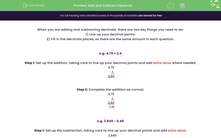### Comprehensive & curriculum aligned

In this worksheet, students will practise adding and subtracting decimals in column method with a need to add zeroes to have the same number of decimal places.Key stage:  KS 4

Year:  GCSE

GCSE Subjects:   Maths

GCSE Boards:   Pearson Edexcel, OCR, Eduqas, AQA,

Curriculum topic:   Number, Fractions, Decimals and Percentages

Curriculum subtopic:   Structure and Calculation Decimal Fractions

Popular topics:   Decimals worksheets

Difficulty level:#### Worksheet Overview

When you are adding and subtracting decimals, there are two key things you need to do:

1) Line up your decimal points.

2) Fill in the decimals places, so there are the same amount in each question.

e.g. 4.75 + 2.4

Step 1: Set up the addition, taking care to line up your decimal points and add extra zeros where needed.

 4.75 +  2.40

Step 2: Complete the addition as normal.

 4.75 +  2.40 7.15

e.g. 3.845 - 0.48

Step 1: Set up the subtraction, taking care to line up your decimal points and add extra zeros.

 3.845 -  0.480

Step 2: Complete the subtraction as normal.

 3.845 -  0.480 3.365

Before you start this activity, grab a pen and paper so you can write out your own addition and subtraction sums to work them out.

### What is EdPlace?

We're your National Curriculum aligned online education content provider helping each child succeed in English, maths and science from year 1 to GCSE. With an EdPlace account you’ll be able to track and measure progress, helping each child achieve their best. We build confidence and attainment by personalising each child’s learning at a level that suits them.

Get started